# Two-dimensional NMR spectroscopy

## The rotating coordinates or rotating frame

It is convenient to figure only the magnetic momentum of the excess of the nuclei in their fundamental states (Fig.3)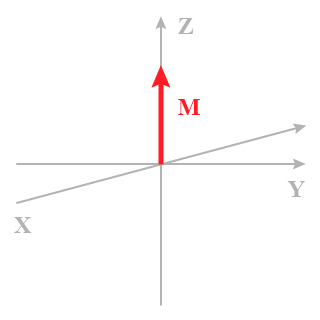Fig. 3 : The magnetisation M in the rotating frame K'

M is the macroscopic magnetization as a result of the individual nuclear moment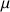. The xy plane does not contain any magnetization component. In addition to the coordinate system K(x,y,z), another system K'(x,y,z) rotates around the z axis with the angular speed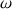thus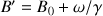. In this figure,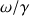is a virtual ﬁeld Bf opposite to B0, which is generated by the only relative rotation from one versus the other of the two coordinate systems K and K'. This means that thevector is static in the system K' which is called rotating frame, it happens whenhas the same sign and the same value than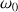.

1. H. Günther

La spectroscopie de RMN MASSON 1994